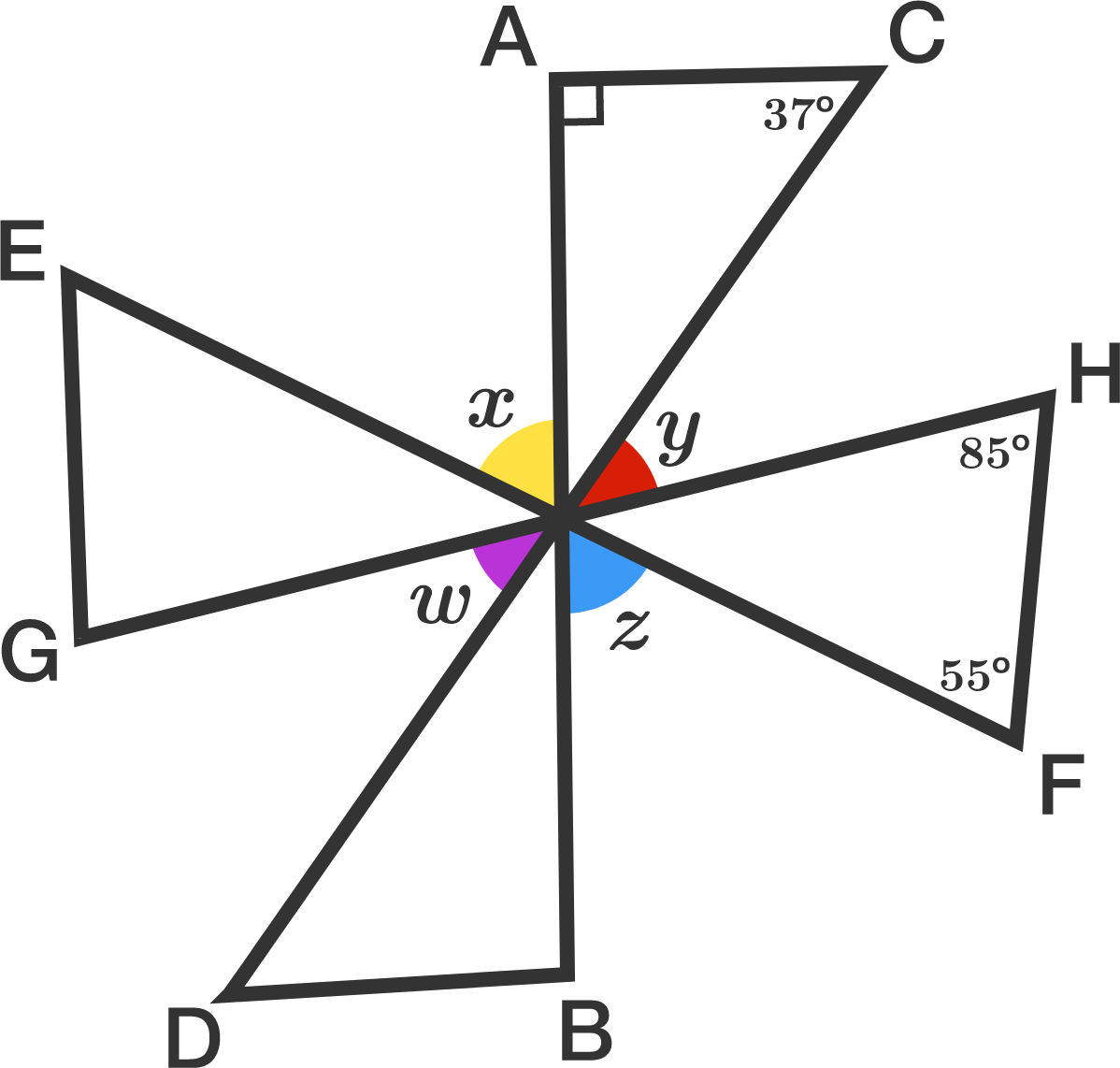# Angles Of A Pinwheel

Geometry Level 1In the figure, four line segments $AB, CD, EF, GH$ intersect at a single point.

Find the value (in degrees) of the angle sum $x + y + w + z.$


Note: $\angle EFH = 55 ^ \circ$, $\angle ACD = 37 ^ \circ$, and $\angle GHF = 85 ^ \circ$.

×

Problem Loading...

Note Loading...

Set Loading...